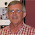## Thursday, September 5, 2013

### ThinkNum - A new interactive public database and graphing tool!

Thinknum.com is a new free data service similar to Quandl.com in that they organize and list publicly available data sets.  Currently they are using data from 2,000 data sources which sounds good compared with Quandl's 400+ source.  Overall, in terms of data points, I don't know which has more.  But that is not really the point.

What it does have however is an advanced user interface in which users can specify functions which they would like to perform analysis on right off of the ThinkNum website.  ThinkNum maintains a high level of credibility in how these functions are implemented in that they list all of the code that enters each of the functions so that there is no behind the curtain ambiguity.

ThinkNum can easily generate a wide range of charts using data names to identify which data sets to use and functions to define how to modify the data sets.  For example:

Say you were interested in how google stock price is moving with respect to the S&P 500.  You want to compare both the daily rate and the 30 day simple moving average and have them plotted on the same graph.  The following command will do this. sma(^spx,30);^spx;sma(goog*2,30);(goog*2); sma is the "simple moving average" while ^spx specifies the  S&P 500 with goog Google and the 30 is the 30 day moving average.  Note, I have multiplied the Google by 2 so that we can see them more clearly on the same graph since the stock price of Google is about half that of the S&P and price scales are arbitrary generally.

function: sma(^spx,30);^spx;sma(goog*2,30);(goog*2);

They have dozens of other functions. You can browse the function library here: http://www.thinknum.com/browseFunctions

In addition, ThinkNum has released an R package called "Thinknum" with the single function "Thinknum" which allows for time series data to be imported directly into R.

install.packages("ThinkNum")

library("Thinknum")
goog <- Thinknum("goog")
plot(goog, type="l")

In order to pass arguments to R in the same manner as the ThinkNum website I have written a small function which can read multiple calls to Thinknum and returns them as either a wide or tall data.frame

mThinknum <- function(command, tall=F) {

require("Thinknum")

# Break the command into seperate calls
think.list <- strsplit(command, ";")[]

# Look through each element of the think.list
for (i in 1:length(think.list)) {

if (!tall) {
if (i==1) returner <- Thinknum(think.list)
if (i>1)  returner <- merge(returner,Thinknum(think.list[i])
, by.x="date_time", by.y="date_time")
}
if (tall) {
tempdat <- Thinknum(think.list[i])
names(tempdat) <- c("date_time", "value")

if (i==1) returner <- data.frame(tempdat, call=think.list[i])
if (i>1) returner <- rbind(returner, data.frame(tempdat, call=think.list[i]))
names(returner) <- c("date_time", "value", "call")
}

cat(paste(rep(" ", max(1,20-nchar(think.list[i]))), collapse=""))
# Insert spaces
cat(paste("Dimensions:", paste(dim(returner), collapse="x"), "\n"))
# Show dimensions
}

# Ensure the return file has appropriate column names
if (!tall) names(returner) <- c("date_time", think.list)

data.frame(returner)
}

test <- mThinknum("^spx;sma(^spx,30);sma(goog*2,30);(goog*2)")

# Let's try plotting with the base package

plot(x=c(min(test\$date_time), max(test\$date_time)),
y=c(min(test[,2:5]),max(test[,2:5])), type="n",
main="Plot in R")

lines(test\$date_time, test[,2], type="l")
lines(test\$date_time, test[,3], type="l", col="red")
lines(test\$date_time, test[,4], type="l", col="blue")
lines(test\$date_time, test[,5], type="l", col="darkgreen")

# Let's do the same but now let's use ggplot2

# In order to do this let's use the tall option for mThinknum

test2 <- mThinknum("^spx;sma(^spx,180);sma(goog*2,180);(goog*2)", tall=T)
# I have extended the running average to be 180 days rather than 30 because the scale is
# so large.

library("ggplot2")

ggplot(data=test2[test2\$date_time>as.Date("2004-01-01"),],
aes(x=date_time, y=value, group=call)) +
geom_line(aes(colour = call))

Created by Pretty R at inside-R.org

Overall, ThinkNum seems to be a pretty cool tool for supplying data and building analytical graphs.  And in addition to all of this, ThinkNum also provides a platform for doing counter-factual data analysis!  This I will not go into since I have not played around with it much.  However, it appears very interesting!  See the below example on how ThinkNum may be used to calculate expected price of Google.  I don't know anything about finance so this is all way over my head.

http://www.thinknum.com/cashflowmodel/?ticker=goog

1.Thanks for sharing this!

2.we have updated our api package to support multiple expressions in one call (like the web gui) so now you can do Thinknum("^spx;sma(^spx,30);sma(goog*2,30);(goog*2)") in your example.

Thanks for the idea and we were super-thrilled to see the post.

Greg, gregory.ugwi@thinknum.com

3.Using Thinknum the time series for ^spx starts at 1950-01-01. But the time series for sma(^spx,30) starts at 1950-02-08. For a 30 day moving average, shouldn't it have started at/around 1950-01-16?